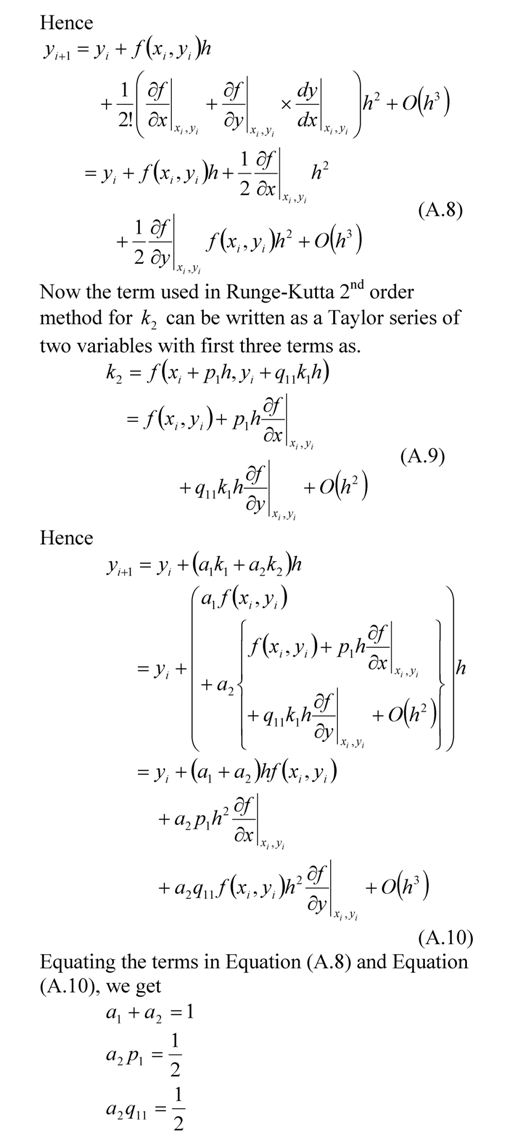# Runge-Kutta 2nd order equations derived

In my class, I present the 2nd order Runge-Kutta method equations without proof. Although I do discuss where the equations come from, there are still students who want to see the proof. So here it is._____________________________________________________

This post is brought to you by Holistic Numerical Methods: Numerical Methods for the STEM undergraduate at http://numericalmethods.eng.usf.edu